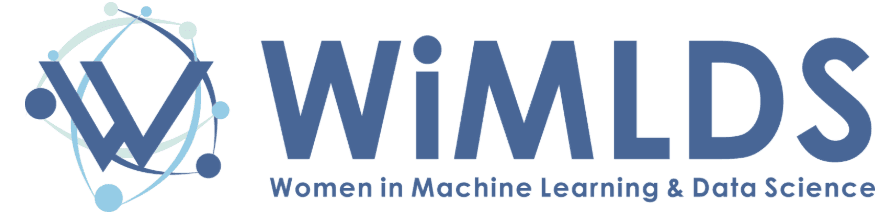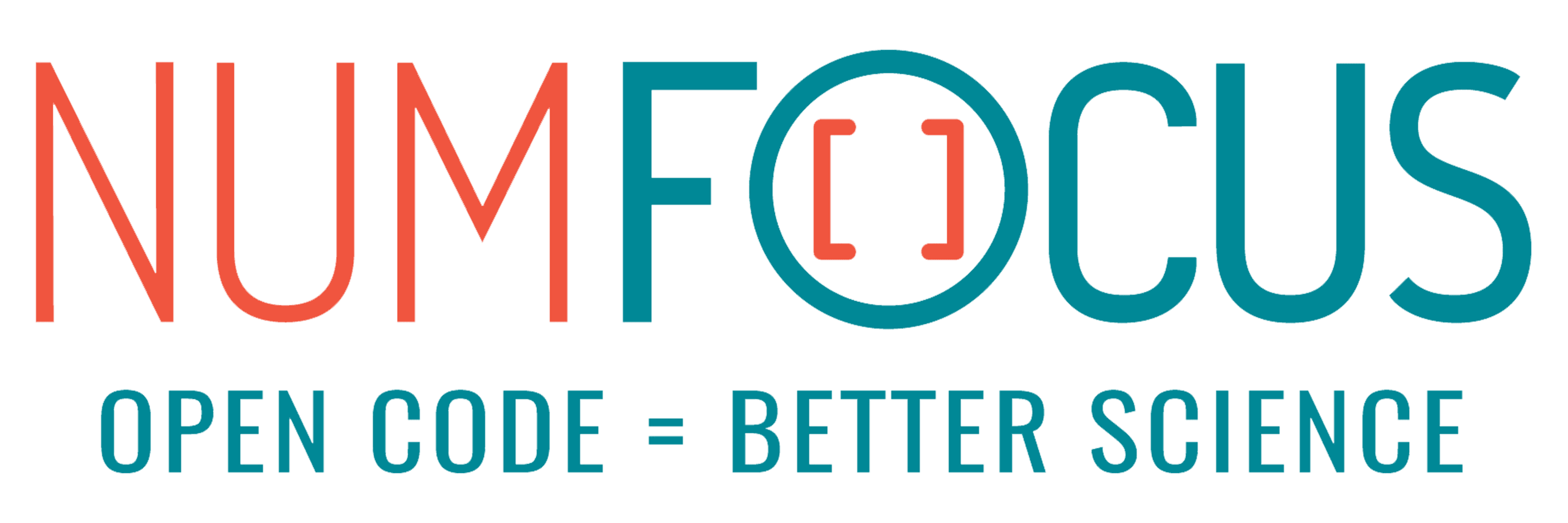# Fast optimization via randomized numerical linear algebra

07/29/2022, 7:30 PM8:00 PM UTC
JuMP

#### Abstract:

We introduce RandomizedPreconditioners.jl, a package for preconditioning linear systems using randomized numerical linear algebra. Crucially, our preconditioners do not require a priori knowledge of structure present in the linear system, making them especially useful for general-purpose algorithms. We demonstrate significant speedups of positive semidefinite linear system solves, which we use to build fast constrained optimization solvers.

#### Description:

In this talk, we discuss how techniques in randomized numerical linear algebra can dramatically speed up linear system solves, which are a fundamental primitive for most constrained optimization algorithms.

We start with randomized approximations of matrices and introduce the Nystrom Sketch. Following the approach developed by Frangella et al. , we use this sketch to construct preconditioners for positive definite linear systems.

We then introduce RandomizedPreconditioners.jl, a lightweight package which includes these randomized preconditioners and sketches. We show how this package allows preconditioners to be added with only a few extra lines of code, and we use the package to dramatically speedup a convex optimization solver that uses the alternating direction method of multipliers (ADMM) algorithm. We demonstrate how we can amortize the cost of this preconditioner over all solver iterations, allowing us to capture this speedup for minimal additional cost

Finally, we conclude with future work to address other types of linear systems and other ways to speed up optimization solvers with randomized numerical linear algebra primitives that are implemented in RandomizedPreconditioners.jl.

 Zachary Frangella, Joel A Tropp, and Madeleine Udell. “Randomized Nyström Preconditioning.” In:arXiv preprint arXiv:2110.02820(2021). https://arxiv.org/abs/2110.02820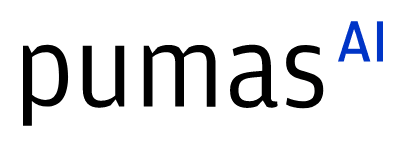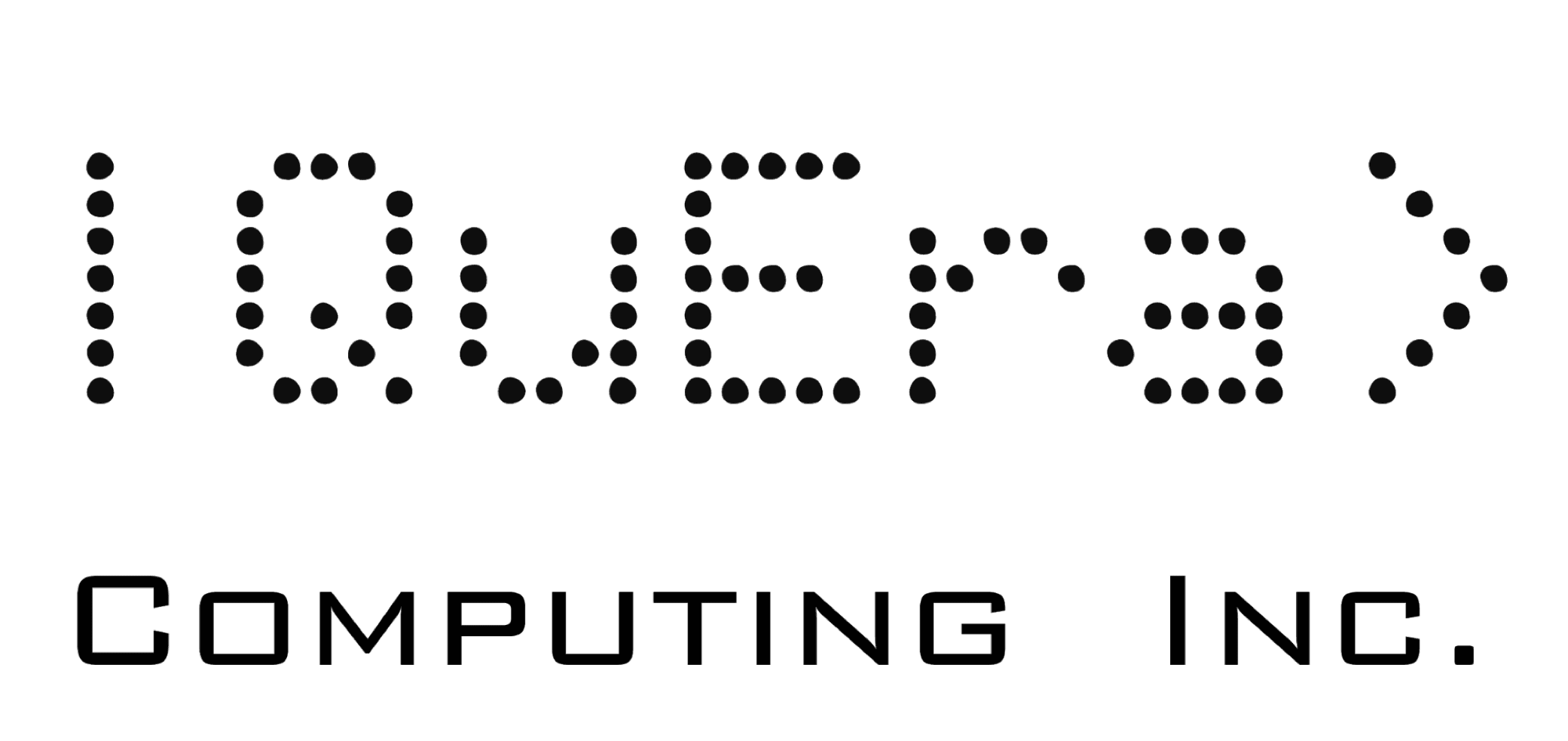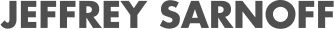## Media partners## Community partners